# Binary option pricing

The payoff of binary options differ from those of regular options. Binary options either have a positive payoff or none. In the case of a binary call, if the price at a certain date, ST,  is larger than or equal to a strike price K, it will generate a payoff Q. Notice, that it does not matter whether the future stock price just equals the strike, is somewhat larger or a lot larger. Thus as long as the stock price is larger than or equal to K, the payoff of a binary does not change.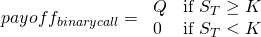The same holds in the case of a binary put. Of course, this option only generates a payoff Q, if the stock price ST, is smaller than the strike price K.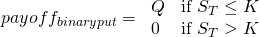Notice that binary option trading is strongly seen as pure speculation and even gambling. Due to the resemblance of the binary option payoff with sports betting, it is hard to justify its hedging value in any risk management exercise.

## Binary option pricing: simulation ingredients

The most straightforward way in pricing a binary option is done through a simulation experiment. In many simulation exercises, the geometric Brownian motion, as shown below, can be used to model the underlying stock behaviour. In this formula S equals the price of the stock, μ equals the stock’s return, σ equals the stock’s volatility and Δt equals 1 time step. Another possibility to value binary options is the construction of a multi-step binomial model.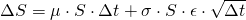In order to implement the stock price evolution in Excel this has to be restated as follows: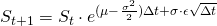With an uncertainty parameter ε generated by a certain distribution, often just a normal distribution.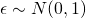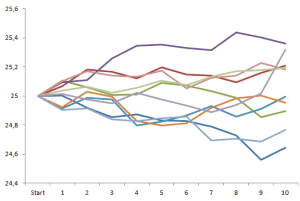## Binary option pricing: simulation implementation

The value of a Binary option can be calculated based on the following method:

Step 1: Determine the return μ, the volatility σ, the risk free rate r, the time horizon T and the time step Δt

Step 2: Generate using the formula a price sequence

Step 3: Calculate the payoff of the binary call and, or put and store it

Step 4: Apply step 2 and 3 N times (e.g. 10000)

Step 5: Calculate the average of all the stored payoffs

Step 6: Discount this value back to today

## Summary

Binary options either generate in the future a certain payoff as specified by the contract or none at all. Binary option pricing can be done through a Monte Carlo simulation experiment. Because of its fixed payoff and its resemblence to sport betting, binary option trading is often seem as pure speculation or gambling.

### Binary option pricing Excel implementation

Need to have more insights? Download our free excel file: binary option pricing.